Next: Multiplication Up: Signed Binary Stream Operations Previous: Signed Binary Stream Operations

## Average

The algorithm for computing the average of two signed binary streams is more complicated than the one for dyadic streams described in section 4.2.1. This is because unlike dyadic digits. individual signed binary digits are not closed under average.

The algorithm described here may require two digits of the input streams to generate one digit of output. A `carry' is used, but the carry is from left (most significant digit) to right (least significant digit), unlike conventional addition algorithms in which the carry occurs from right to left.

We describe the algorithm by first developing it from scratch and the proving its correctness. We then summarise the development by expressing the algorithm as a recursive function which computes the average of two inputs.

Suppose we wish to compute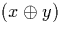where x is of the form (a0::a1::x'') and y is of the form (b0::b1::y''). We use signed binary carry digit c, which adds c/2 to the number represented by the result: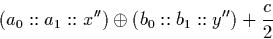Using the properties described in section 3.6.2, this is equal to:

We now wish to generate one signed binary digit of output, and express the remainder of the number as a recursive call. Let e be the digit which is to be output, and the amount c'/2 be the amount which cannot be represented exactly in the digit e and is to be added to the remainder of the stream by passing the digit c' right as the new carry.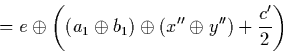These equations may be expanded to give: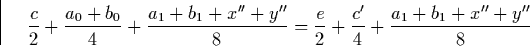By re-arranging this we get c' = a0 + b0 + 2c - 2e.

Now let us assume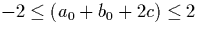. We now show how to compute e and c' so that this assumption will hold when we make the recursive call to the stream average operation. Observe that as c = 0 initially, the assumption holds. Now find e, c' such that

As, we find e as follows. Let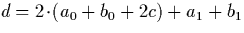: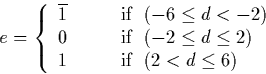These equations lead to an algorithm for average, although it is more complex than dyadic average. In certain situations, however, it is not necessary to examine two digits of each input stream as the average of the first input digits and carry digit may be expressed exactly as a signed binary digit with a carry of zero.

The algorithm developed and proved above amounts to the following recursive definition.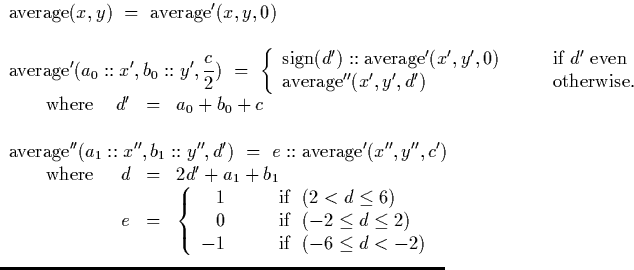The sign function is defined as follows: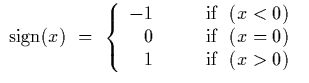The implementation of this algorithm is described in section 6.3, and its behaviour is examined further in section 7.1.2.Next: Multiplication Up: Signed Binary Stream Operations Previous: Signed Binary Stream Operations
Martin Escardo
5/11/2000